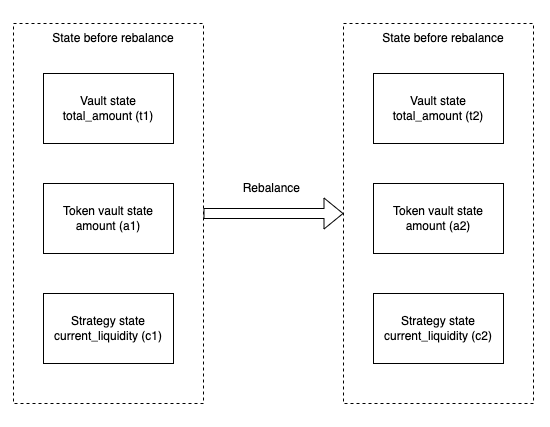# Rebalance crank

When an operator submits tx deposit_strategy or withdraw_strategy, we call this the rebalance crank.We call state variables before the rebalance:
• vault.total_amount : t1
• token_vault.amount : a1
• strategy.current_liquidity : c1
And the state variables after the rebalance:
• vault.total_amount : t2
• token_vault.amount : a2
• strategy.current_liquidity : c2
Then the vault would know that the total accrued interest after rebalance is:
$profit = (c2+a2) - (c1+a1)$
Then vault can update the total amount:
$t2 =t1 +profit$
Or:
$t2 =t1 + (c2+a2) - (c1+a1)$
The immediate release of profit generated to the LPs can result in opportunities for a sandwich attack as seen in the scenario below:An attacker is able to time their deposit before a rebalance and withdraw immediately after a rebalance in order to get the max profit. In the example above, the attacker is able to earn 10 tokens as a reward by executing the sandwich attack.

### Sandwich Attack Prevention

Instead of distributing 100% of the yield generated by the lending platforms to the LPs immediately after rebalancing, the system will drip the yield to the LPs across a pre-determined period of time.
We need to define the following state variables Total_amount: The total amount of liquidity collected (balance in vault + balances in all strategies) Last_updated_locked_profit: Total locked profit, updated every time we do rebalancing Last_report: timestamp of the last rebalancing run Locked_profit_degradation: Rate at which the profit will be unlocked in % per second e.g. 0.1% of locked_profits released per second.
When a user adds or removes liquidity, instead of using vault.total_amount to calculate the total lp to mint or burn, we use the get_unlock_amount function to calculate the unlocked_amount value to use.
$duration=currentTime - lastReport$
$lockedFundRatio=1 - duration * lockedProfitDegradation$
$unlockedAmount=totalAmount - lastUpdatedLockedProfit * lockedFundRatio$
Example: Drip duration: 5 minsAs seen in the example above, the rebalancing at t = 2 mins will gain a total of 100 token as yield from the lending platforms. However, as we are using the unlocked amount value to calculate the withdrawal amount, the attacker will not get any of the yield gained if he were to withdraw his tokens immediately after rebalancing. He will also only gain a fraction of the total yield if he withdraws within the next few minutes.